Next: Curvilinear Coordinates Up: Vector Algebra and Vector Previous: Gradient

It is useful to define the vector operator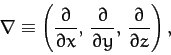(1362)

which is usually called the grad or del operator. This operator acts on everything to its right in a expression, until the end of the expression or a closing bracket is reached. For instance,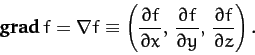(1363)

For two scalar fields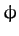and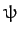,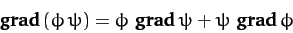(1364)

can be written more succinctly as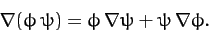(1365)

Suppose that we rotate the coordinate axes through an angleabout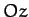. By analogy with Equations (A.1277)-(A.1279), the old coordinates (,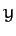,) are related to the new ones (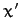,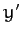,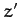) via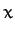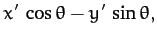(1366)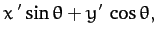(1367)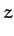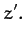(1368)

Now,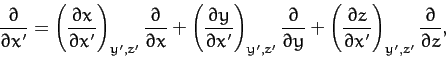(1369)

giving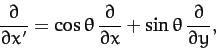(1370)

and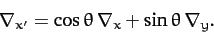(1371)

It can be seen, from Equations (A.1280)-(A.1282), that the differential operator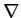transforms in an analogous manner to a vector. This is another proof that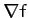is a good vector.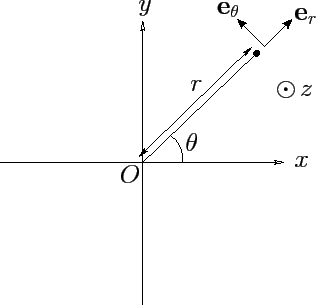Next: Curvilinear Coordinates Up: Vector Algebra and Vector Previous: Gradient
Richard Fitzpatrick 2011-03-31